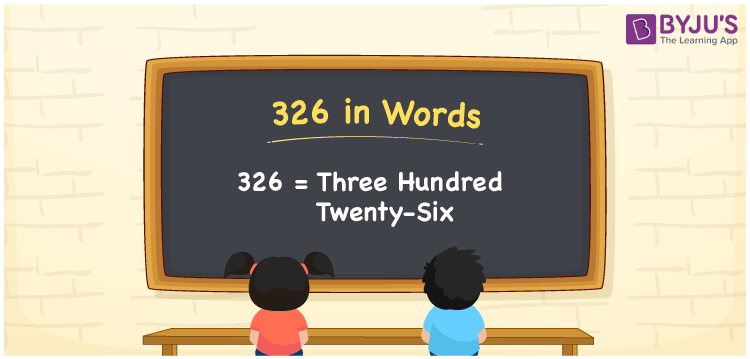# 326 in Words

326 in words is written as Three hundred twenty-six. In both the International System of Numerals and the Indian System of Numerals, 326 is written as Three hundred twenty-six. The number 326 is a Cardinal Number as it represents some quantity. For example, “there are 326 chairs in the room”.

 326 in Words Three hundred twenty-six Three hundred twenty-six in Number 326

## 326 in English Words

We write 326 in English Words using the letters of the English alphabet. Therefore, we read 326 in English as “Three hundred twenty-six.”## How to Write 326 in Words?

To write 326 in words, we shall use the place value chart. In the place value chart, write 3 in the hundreds, 2 in the tens, and 6 in the ones, respectively. Now let us make a place value chart to write the number 326 in words.

 Hundreds Tens Ones 3 2 6

Thus, we can write the expanded form as

3 × Hundred + 2 × Ten + 6× One

= 3 × 100 + 2× 10 + 6× 1

= 300 + 20+ 6

= 326

= Three hundred twenty-six.

326 is a natural number, the successor of 325 and the predecessor of 327.

326 in words – Three hundred twenty-six

• Is 326 an odd number? – Yes
• Is 326 an even number? – No
• Is 326 a perfect square number? – No
• Is 326 a perfect cube number? – No
• Is 326 a prime number? – No
• Is 326 a composite number? – Yes

## Frequently Asked Questions on 326 in Words

### How to write 326 in words?

326 in words is written as Three hundred twenty-six.

### How to write 326 in the International and Indian System of Numerals?

In both, the system of numerals, 326 in words, is written as Three hundred twenty-six.

### What is the preceding number of 326?

The number that precedes 326 is 325.